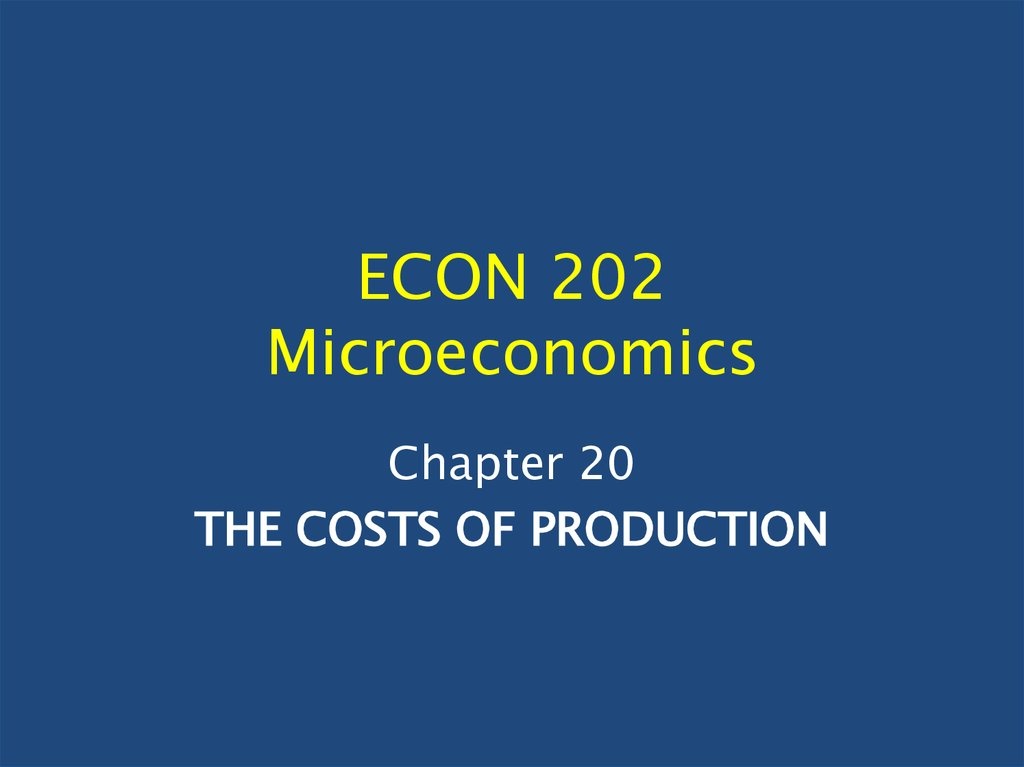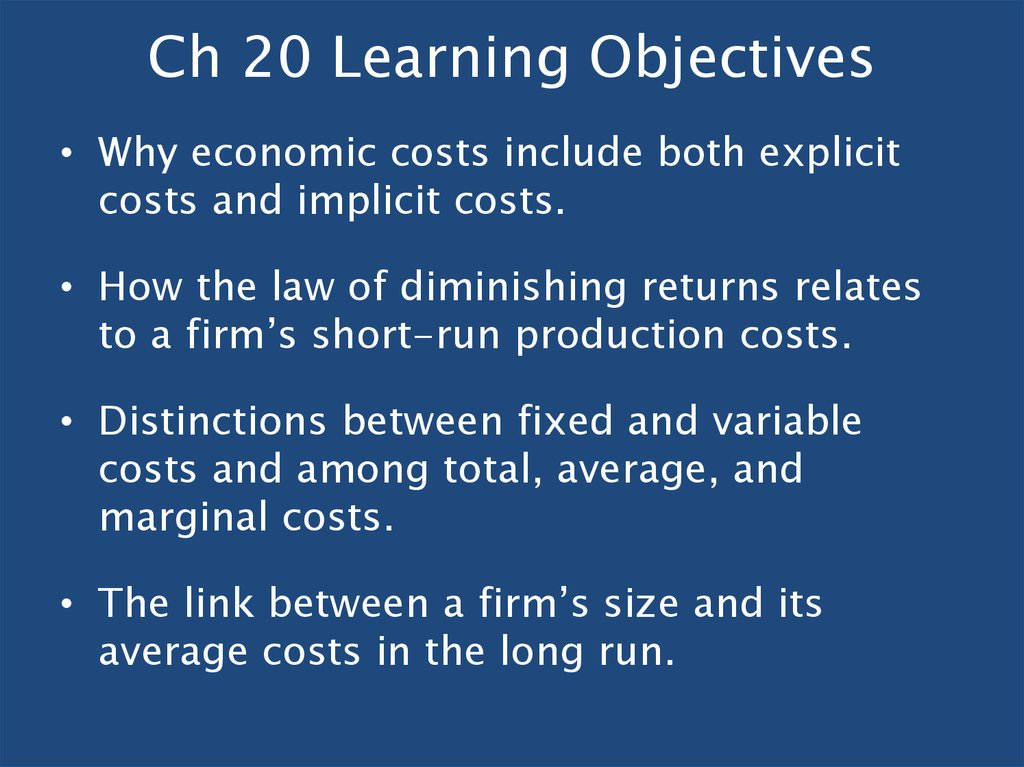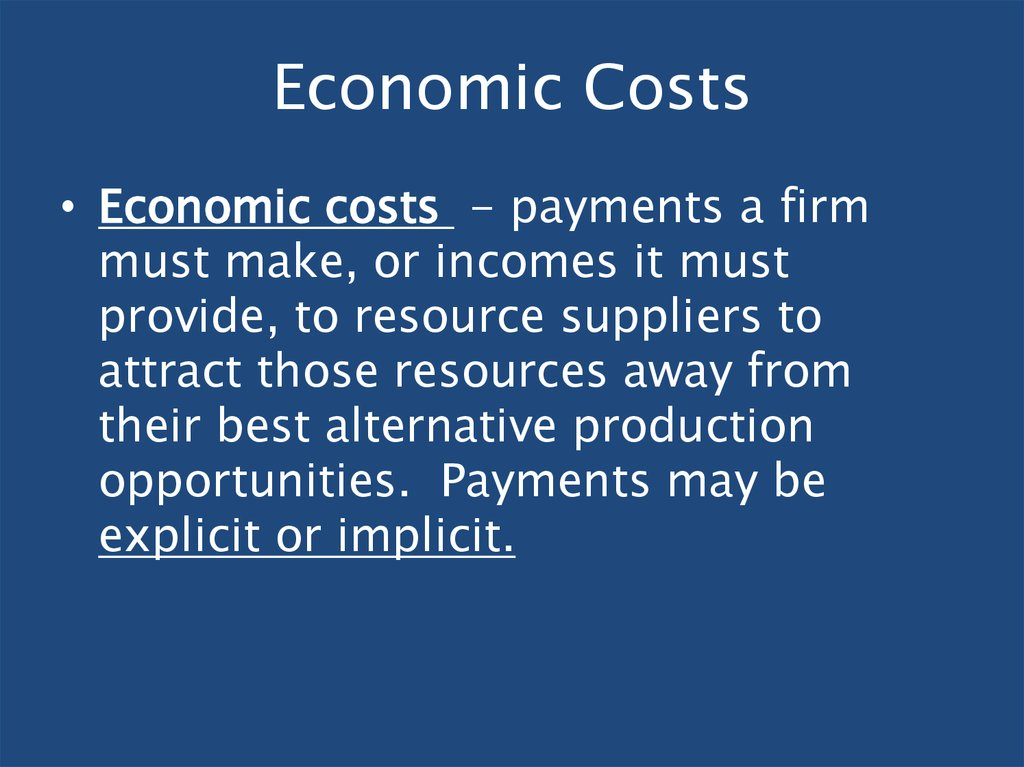# Microeconomics. The costs of production. Chapter 20

## 1. ECON 202 Microeconomics

Chapter 20
THE COSTS OF PRODUCTION

## 2. Ch 20 Learning Objectives

• Why economic costs include both explicit
costs and implicit costs.
• How the law of diminishing returns relates
to a firm’s short-run production costs.
• Distinctions between fixed and variable
costs and among total, average, and
marginal costs.
• The link between a firm’s size and its
average costs in the long run.

## 3. Economic Costs

• Economic costs - payments a firm
must make, or incomes it must
provide, to resource suppliers to
attract those resources away from
their best alternative production
opportunities. Payments may be
explicit or implicit.

## 4. Explicit Costs

• Cash Payments a firm makes to those
who supply labor services, materials,
fuel, transportation services, etc.
• Money payments are for the use of
resources owned by others.

## 5. Implicit Costs

• Implicit costs - opportunity costs of
using its self-owned, self-employed
resources.
• Money payments that self-employed
resources could have earned in their
best alternative use
• Forgone interest, forgone rent,
forgone wages, and forgone
entrepreneurial income.

## 6.

• T-shirts example: Accounting profits
- \$57,000
• Ignores implicit costs
• Overstates economic success

## 7. Normal Profits

• Normal profits are considered an
implicit cost because they are the
minimum payments required to keep
the owner’s entrepreneurial abilities
self-employed. This is \$5,000 in the
example.
• Cost of doing buisiness

## 8. Economic Profits

• Economic or pure profits are total
revenue less all costs (explicit and
implicit including a normal profit).

## 9. Short Run

• Time period that is too brief for a firm
to alter its plant capacity. The plant
size is fixed in the short run.
• Short-run costs, then, are the wages,
raw materials, etc., used for
production in a fixed plant.

## 10. Long-run

• The long run is a period of time long
enough for a firm to change the
quantities of all resources employed,
including the plant size.
• Long-run costs are all costs, including
the cost of varying the size of the
production plant.

## 11.

Economic Profit Versus Accounting Profits
Economic
Profit
Implicit Costs
(Including a
Normal Profit)
Explicit
Costs
Accounting
Total Revenue
Economic
(Opportunity)
Costs
Economics
Accounting
Profit
Accounting
Costs (Explicit
Costs Only)
Short Run and Long Run
•Short Run: Fixed Plant
•Long Run: Variable Plant

## 12. Short-Run Production Relationships

• Total Product (TP)
• Marginal Product (MP)
• Average Product (AP)
Change in Total Product
Marginal Product =
Change in Labor Input
Average Product
=
Total Product
Units of Labor
W 20.2

## 13. Law of Diminishing returns

• Assumes technology is fixed & techniques
for production do not change.
• As successive units of a variable resource
are added to a fixed resource, beyond some
point the extra or marginal product that can
be attributed to each additional unit of the
variable resource will decline.

## 14. Law of Diminishing Returns

(1)
Units of the
(2)
Variable Resource Total Product
(Labor)
(TP)
0
0
]
10
1
]
25
2
]
45
3
]
60
4
]
70
5
]
75
6
]
75
7
]
70
8
(3)
Marginal Product
(MP),
Change in (2)/
Change in (1)
10
15
20
15
10
5
0
-5
(3)
Average
Product
(AP),
(2)/(1)
Increasing
10.00
Marginal
12.50
Returns
15.00
Diminishing
15.00
Marginal
14.00
Returns
12.50
Negative
10.71
Marginal
Returns
8.75

## 15. Law of Diminishing Returns

Total Product, TP
• Graphical Portrayal
30
20
10
0
Marginal Product, MP
TP
1
2
3
Increasing
Marginal
20 Returns
4
5
6
7
8
9
Negative
Marginal
Returns
Diminishing
Marginal
Returns
10
AP
1
2
3
4
5
6
7
8 9
MP

## 16. Law of Diminishing Returns Example

• For example, a farmer will find that a
certain number of farm laborers will
yield the maximum output per worker.
If that number is exceeded, the output
per worker will fall.
• Table 20.1 - Example of output per
labor unit.

## 17.

• The law of diminishing returns
assumes all units of variable inputs—
workers in this case—are of equal
quality. Marginal product diminishes
not because successive workers are
inferior but because more workers are
being used relative to the amount of
plant and equipment available.

• Fixed Costs
• Variable Costs
• Total Cost
TC = TFC + TVC

## 19. Short-Run Production Relationships

• Short-run production reflects the law
of diminishing returns that states that
as successive units of a variable
resource are added to a fixed
resource, beyond some point the
resource unit will decline.

## 20. Short Run Production Costs

• Fixed, variable and total costs
– 1. Total fixed costs are those costs whose total does not
vary with changes in short-run output.
– 2. Total variable costs are those costs that change with
the level of output. They include payment for materials,
fuel, power, transportation services, most labor, and
similar costs.
– 3. Total cost is the sum of total fixed and total variable
costs at each level of output (see Figure 20.3).

## 21. Short Run Production Costs

• Per unit or average
– 1. Average fixed cost is the total fixed cost divided by the
level of output (TFC/Q). It will decline as output rises.
– 2. Average variable cost is the total variable cost divided
by the level of output (AVC = TVC/Q).
– 3. Average total cost is the total cost divided by the level
of output (ATC = TC/Q), sometimes called unit cost or
per unit cost. Note that ATC also equals AFC + AVC (see
Figure 20.4).

## 22. Short Run Production Costs

• Marginal cost - additional cost of producing one more unit of
output (MC = change in TC/change in Q).
– 1. Marginal cost can also be calculated as MC = change in TVC/change
in Q.
– 2. Marginal decisions are very important in determining profit levels.
Marginal revenue and marginal cost are compared.
– 3. Marginal cost is a reflection of marginal product and diminishing
returns. When diminishing returns begin, the marginal cost will begin
its rise.
– 4. The marginal cost is related to AVC and ATC. These average costs will
fall as long as the marginal cost is less than either average cost. As soon
as the marginal cost rises above the average, the average will begin to rise.
Students can think of their grade-point averages with the total GPA
reflecting their performance over their years in school, and their marginal
grade points as their performance this semester. If their overall GPA is a
3.0, and this semester they earn a 4.0, their overall average will rise, but
not as high as the marginal rate from this semester.

## 23. Short Run Production Costs

• Cost curves will shift if the resource
prices change or if technology or
efficiency change.

## 24. Short-Run Production Costs

• Per-Unit or Average Costs
– Average Fixed Cost (AFC)
– Average Variable Cost (AVC)
– Average Total Cost (ATC)
– Marginal Cost (MC)
TFC
TVC
AFC =
AVC =
Q
Q
TC
= AFC + AVC
ATC =
Q
Change in TC
MC =
Change in Q

## 25. Short-Run Production Costs

Total Cost, Fixed and Variable Costs
\$1100
TC
1000
900
TVC
800
Costs
700
600
Fixed
Cost
500
400
Total
Cost
300
Variable
Cost
200
100
TFC
0
1
2
3
4
5
6
7
8
9
10
Q

## 26. Short-Run Production Costs

Average and Marginal Costs
\$200
MC
Costs
150
AFC
ATC
AVC
100
50
AVC
AFC
0
1
2
3
4
5
6
7
8
9
10
Q
G 20.1

## 27. Short-Run Production Costs

MC and Marginal Product
Marginal Decisions
Relation of MC to AVC and ATC
Relationship Between Productivity
Curves and Cost Curves
• Shifts in Cost Curves
Graphically…

## 28. Short-Run Production Costs

Average Product and
Marginal Product
Production Curves
Cost Curves
AP
MP
Quantity of Labor
MC
Cost (Dollars)
AVC
Quantity of Output

## 29. Long-run

• In the long-run, all production costs
are variable, i.e., long-run costs
reflect changes in plant size and
industry size can be changed (expand
or contract).
• Can change inputs and plant size.

## 30. Economies of Scale

• a.k.a. Economies of mass production
• As plant size increases, a number of
factors will for a time lead to lower
average costs of production.
– Labor Specialization
– Managerial Specialization
– Efficient Capital
– Other Factors

## 31. Diseconomies of Scale

• Over time, thee expansion of a firm
may lead to diseconomies of scale and
therefore higher average total costs.
– Cause – difficulty of efficiency controlling
& coordinating a firm’s operations as it
becomes large.

## 32.

• Economies or diseconomies of scale
exist in the long run.
1. Economies of scale or economies of mass
production explain the downward sloping part of
the long-run ATC curve, i.e. as plant size
increases, long-run ATC decrease.
a. Labor and managerial specialization is one
reason for this.
b. Ability to purchase and use more efficient
capital goods also may explain economies of
scale.
C. Other factors may also be involved, such as
design, development, or other “start up” costs
such as advertising and “learning by doing

## 33. Long-Run Production Costs

• Firm Size and Costs
• Long-Run Cost Curve
• Economies of Scale
– Labor Specialization
– Managerial Specialization
– Efficient Capital
• Diseconomies of Scale
• Constant Returns to Scale

## 34. Long-Run Production Costs

Average Total Costs
Long-Run ATC Curve
ATC-1
ATC-5
ATC-2
ATC-3
ATC-4
Output
Any Number of Short-Run Optimum
Size Cost Curves Can Be Constructed

## 35. Long-Run Production Costs

Average Total Costs
Long-Run ATC Curve
ATC-1
ATC-5
ATC-2
ATC-3
ATC-4
Long-Run
ATC
Output
The Long-Run ATC Curve Just
“Envelopes” the Short Run ATCs

## 36. Long-Run Production Costs

Average Total Costs
Alternative Long-Run ATC Shapes
Constant Returns
To Scale
Economies
Of Scale
Diseconomies
Of Scale
Long-Run
ATC
q1
q2
Output
Long-Run ATC Curve Where Economies
Of Scale Exist

## 37. Long-Run Production Costs

Average Total Costs
Alternative Long-Run ATC Shapes
Economies
Of Scale
Diseconomies
Of Scale
Long-Run
ATC
Output
Long-Run ATC Curve Where Costs Are
Lowest Only When Large Numbers Are
Participating

## 38. Long-Run Production Costs

Average Total Costs
Alternative Long-Run ATC Shapes
Economies
Of Scale
Diseconomies
Of Scale
Long-Run
ATC
Output
Long-Run ATC Curve Where Economies
Of Scale Exist, are Exhausted Quickly,
And Turn Back Up Substantially

## 39. Minimum Efficient Scale and Industry Structure

• Minimum Efficient Scale (MES)
• Natural Monopoly
• Applications and Illustrations

Rising Cost of Insurance and Security
Successful Start-Up Firms
The Verson Stamping Machine
The Daily Newspaper
Aircraft and Concrete Plants

## 40. Don’t Cry Over Sunk Costs

• Sunk Costs Irrelevant in Decision
Making
• Once Incurred, They Cannot Be
Recovered
• Compare Marginal Analysis to Find
MC and MB
• Previously Incurred Costs Do Not
Impact the MB=MC Decision
• Sunk Costs Are Irrelevant!